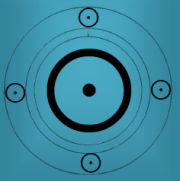November 2015 Paris attacks

21:17 – First suicide bombing near the Stade de France

On 13 November 2015 at 21:17 as TransittSU = AC/MA = MA/PL tME(AC Lord) = ME = MA/SA tMO = SA tSA = tPL = AC/ME(AC Lord) AC is Libra

On 13 November 2015 at 21:17pSU = tSU = AC/MA = MA/PL pAC = tME = pJU = pSA = ME = UR = MA/SA pMC = AC/UR pPL = MO pMO = MN pMA = ME/MA

On 13 November 2015 at 21:17pMA = SU/MA = MC/MA = AC pMN = UR = ME = MA/SA pMO = pPL = AC/ME(AC Lord) pUR = SU pME = SA

SolarNew Moon on 11.11.2015 at 18:47:08 Paris as transittME(ac Lord) = tPL = AC/MA = MA/PL tAC = tUR = UR = ME = MA/SA New Moon = MO tMC = tMA = AC/ME(AC Lord)